For paraxial approximant, we get the following equation:here a =2k0nre f  ,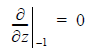From Equation 119, we getand therefore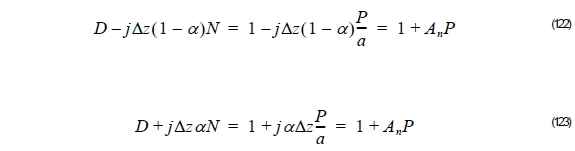whereThus, the unknown at field φ l + 1  at z + Δz is related to the known field φ l  at z as follows: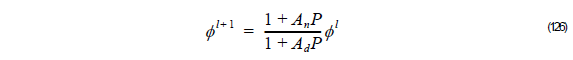or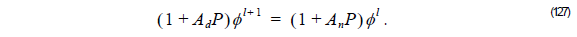Using Equation 127 we finally can get the unknown field φ l + 1  at z + Δz from the known field φ l

If we consider FEM we get,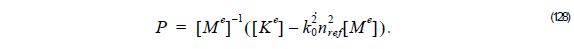Thus, we can rewrite Equation 127 as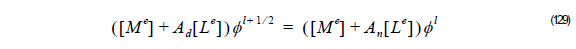here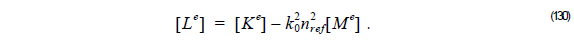The element matrices [ M e] and [ K e] are defined by Equation 100 and Equation 99, respectively.